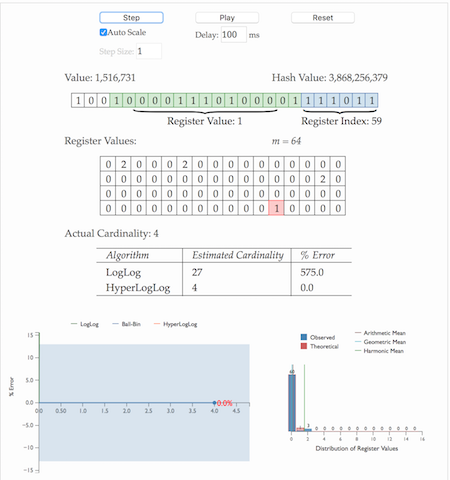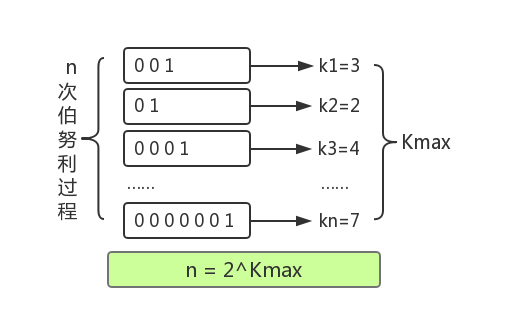# 神奇的HyperLogLog算法

2114℃

## 基数计数基本概念

$S_u$

Su，当新来一个元素

$x_i$

，若

$S_u$

$x_i$

，则将

$x_i$

$S_u$

，否则不加入，计数值就是

$S_u$

1. 当统计的数据量变大时，相应的存储内存也会线性增长
2. 当集合
$S_u$

变大，判断其是否包含新加入元素

$x_i$

的成本变大

### B树

B树最大的优势是插入和查找效率很高，如果用B树存储要统计的数据，可以快速判断新来的数据是否已经存在，并快速将元素插入B树。要计算基数值，只需要计算B树的节点个数。 将B树结构维护到内存中，可以快速统计和计算，但依然存在问题，B树结构只是加快了查找和插入效率，并没有节省存储内存。例如要同时统计几万个链接的UV，每个链接的访问量都很大，如果把这些数据都维护到内存中，实在是够呛。

### bitmap

$001101001$

$[2,3,5,8]$

。新加入一个元素，只需要将已有的bit数组和新加入的数字做按位或

$(or)$

bitmap有一个很明显的优势是可以轻松合并多个统计结果，只需要对多个结果求异或就可以。也可以大大减少存储内存，可以做个简单的计算，如果要统计1亿个数据的基数值，大约需要内存： 100000000/8/1024/1024

$\approx$

12M

32*100000000/8/1024/1024

$\approx$

381M

bitmap对于内存的节约量是显而易见的，但还是不够。统计一个对象的基数值需要12M，如果统计10000个对象，就需要将近120G了，同样不能广泛用于大数据场景。

### 概率算法

• Linear Counting(LC)：早期的基数估计算法，LC在空间复杂度方面并不算优秀，实际上LC的空间复杂度与上文中简单bitmap方法是一样的（但是有个常数项级别的降低），都是
$O(N_{max})$

• LogLog Counting(LLC)：LogLog Counting相比于LC更加节省内存，空间复杂度只有
$O(log_2(log_2(N_{max})))$

• HyperLogLog Counting(HLL)：HyperLogLog Counting是基于LLC的优化和改进，在同样空间复杂度情况下，能够比LLC的基数估计误差更小。

#### HyperLogLog的惊人表现

$2^{64}$

$m$

$S$

，将待统计的数据集合划分成

$m$

$m$

$m$1. 通过hash函数计算输入值对应的比特串
2. 比特串的低
$t(t=log_2^m)$

位对应的数字用来找到数组

$S$

中对应的位置

$i$

3. $t+1$

位开始找到第一个1出现的位置

$k$

，将

$k$

记入数组

$S_i$

位置

4. 基于数组
$S$

记录的所有数据的统计值，计算整体的基数值，计算公式可以简单表示为：

$\hat{n}=f(S)$

1. 为什么要记录第一个1出现的位置？
2. 为什么要有分桶数组
$S$

？

3. 通过分桶数组
$S$

计算基数的公式是什么？

## hyperloglog原理理解

$k$

，将这种抛硬币多次直到出现正面的过程记为一次伯努利过程，对于

$n$

$n$

$k_1$

,

$k_2$

……

$k_n$

，其中最大值记为

$k_{max}$

，那么可以得到下面结论：

1. $n$

次伯努利过程的投掷次数都不大于

$k_{max}$

2. $n$

次伯努利过程，至少有一次投掷次数等于

$k_{max}$

$n$

$k_{max}$

$P_n(X \le k_{max})=(1-1/2^{k_{max}})^n$

$k_{max}$

$P_n(X \ge k_{max})=1-(1-1/2^{k_{max}-1})^n$

$n\ll 2^{k_{max}}$

$P_n(X \ge k_{max})\approx0$

，即当

$n$

$2^{k_{max}}$

$n\gg 2^{k_{max}}$

$P_n(X \le k_{max})\approx0$

，即当

$n$

$2^{k_{max}}$

$2^{k_{max}}$

$n$

$n$

$k$

，那么可以用n次实验中最大的抛掷次数

$k_{max}$

$n$

：

$\hat{n} = 2^{k_{max}}$可以通过一组小实验验证一下这种估计方法是否基本合理。

$k$

，那么基于上面的结论，我们可以通过多次抛硬币实验的最大抛到正面的次数来预估总共进行了多少次实验，同样可以可以通过第一个1出现位置的最大值

$k_{max}$

$k$

，就可以通过其中最大值

${k_{max}}$

$\hat{n} = 2^{k_{max}}$

## hyperloglog算法讲解

### 分桶平均

HLL的基本思想是利用集合中数字的比特串第一个1出现位置的最大值来预估整体基数，但是这种预估方法存在较大误差，为了改善误差情况，HLL中引入分桶平均的概念。

$m$

${k_{max}}$

$\hat{n}$

，最终对这些

$\hat{n}$

求平均得到整体的基数估计值。LLC中使用几何平均数预估整体的基数值，但是当统计数据量较小时误差较大；HLL在LLC基础上做了改进，采用调和平均数，调和平均数的优点是可以过滤掉不健康的统计值，具体的计算公式为：

### 偏差修正

m = 2^b   # with b in [4...16]if m == 16:
alpha = 0.673elif m == 32:
alpha = 0.697elif m == 64:
alpha = 0.709else:
alpha = 0.7213/(1 + 1.079/m)registers = *m   # initialize m registers to 0############################################################################ Construct the HLL structurefor h in hashed(data):
register_index = 1 + get_register_index( h,b ) # binary address of the rightmost b bits
run_length = run_of_zeros( h,b ) # length of the run of zeroes starting at bit b+1
registers[ register_index ] = max( registers[ register_index ], run_length )########################################################################### Determine the cardinalityDV_est = alpha * m^2 * 1/sum( 2^ -register )  # the DV estimateif DV_est < 5/2 * m: # small range correction
V = count_of_zero_registers( registers ) # the number of registers equal to zero
if V == 0:  # if none of the registers are empty, use the HLL estimate
DV = DV_est
else:
DV = m * log(m/V)  # i.e. balls and bins correctionif DV_est <= ( 1/30 * 2^32 ):  # intermediate range, no correction
DV = DV_estif DV_est > ( 1/30 * 2^32 ):  # large range correction
DV = -2^32 * log( 1 - DV_est/2^32)

## redis中hyperloglog实现

redis正是基于以上的HLL算法实现的HyperLogLog结构，用于统计一组数据集合中不重复的数据个数。 redis中统计数组大小设置为，hash函数生成64位bit数组，其中位用来找到统计数组的位置，剩下50位用来记录第一个1出现的位置，最大位置为50，需要 位记录。